This article was first published on OSM , and kindly contributed to python-bloggers. (You can report issue about the content on this page here)

In our last post, we explored mean-variance optimization (MVO) and finally reached the efficient frontier. In the process, we found that different return estimates yielded different frontiers both retrospectively and prospectively. We also introduced the concept of satsificing, originally developed by Herbert Simon. Simply put, satisficing is choosing the best available solution afforded by a messy reality, when an optimal solution requires hard to know (or unknowable) information. Alternatively, an optimal solution might be easy to find, but requires conditions so abstracted from normal phenomena as to be devoid of reality.

Indeed, as we saw in a few examples, the optimal portfolio weights in one period, were not so in another. If the “optimal” solution to portfolio allocation is a moving target, and has a hard time dealing with the noisiness of financial data, then it’s easy to understand the appeal of “good-enough” models like the 1/n or 60/40 stock/bond portfolio.

Yet, are those good enough models, good enough? We’ll try to answer that question in this post, where we’ll compare MVO allocations to our satisfactory portfolio from I like to MVO it! and the 1/n allocations. Let’s begin.

The most naive portfolio allocation is the “1/n”, called such because it allocates to each asset an amount equal to the inverse of the number of assets (n) in the portfolio. Calling it the equal-weighted portfolio seems a bit more in line with its naivete. But that’s finance for you. In any case, this is how we plan to compare the MVO allocations with the 1/n and our satisfactory portfolio. We’ll use the return simulations we created in Testing expectations as our laboratory. For each simulation we’ll calculate the returns, risk, and Sharpe ratios using weightings from our four target portfolio allocations. Then we’ll compare the metric of interest—average or risk-adjusted return—and the frequency of that performance.

Before we do this, however, we need to be clear about which part of the efficient frontier we’re using. Typically, finance folks look at the portfolio with the lowest risk (i.e., “minimum variance”) or the best risk-adjusted return (e.g., the maximum Sharpe or “tangency”).1 However, most people are more concerned with the highest returns or risk-adjusted returns. Hence, we’ll focus on those portfolios on the efficient frontier.

To get a sense of what’s going on behind the scenes we’ll show you a representative graph. For the graph, we calculate the range of portfolios using our weighting algorithm on one of the simulations. We also calculate the returns and risk for that simulation using our satisfactory portfolio weights along with the weights based on the MVO and 1/n portfolios. Recall, the MVO weights were originally calculated using returns from the five-year the period of 1987-1991. The return simulations were calculated using most of the concurrent data available from 1971-1991.The satisfactory, naive, maximal Sharpe ratio efficient, and maximal return efficient portfolios are colored in blue, black, red and purple.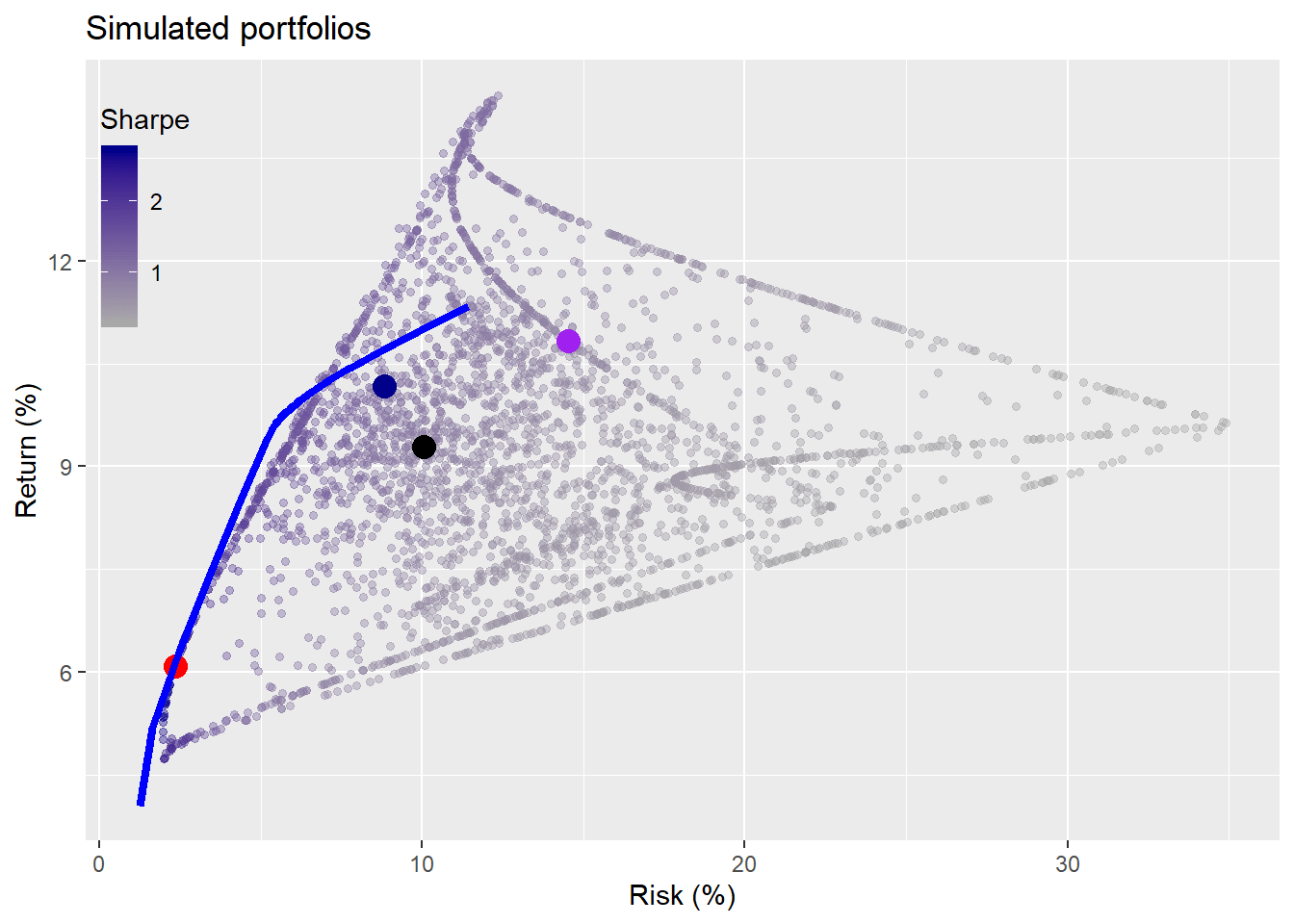Doesn’t look like the MVO portfolios are that optimal out-of-sample. We promise we didn’t cherry pick the simulation. Whatever the case, this is only one instance out of a thousand, so let’s get into the meat of the analysis.

We run the first round of portfolio results to see how the different allocation regimes perform. First, we look at average returns for the highest MVO-derived Sharpe ratio (“Sharpe”), satisfactory, naive, and maximum MVO-derived return (“Max”) portfolios.The highest Sharpe ratio portfolio underperforms the others, while the maximum return portfolio does what it says on the tin. This is an interesting result for the following reason. The maximum return portfolio was constructed on backward-looking data and didn’t cover the full period in which we had data. Nevertheless, it produced a higher average return on 1,000 out-of sample simulations compared to the others. No wonder Markowitz won the Nobel Prize in Economics! Still, we’ll need to look at its performance compared to all portfolios later in the post. Moreover, there might be some statistical issues with our simulations—sampling from a normal distribution when returns are not normally distributed for instance. Additionally, even though the simulations used a longer time series than the one used to calculate the efficient frontier, we assume there wasn’t a statistically significant difference in return and risk between the series. We’d, of course, have to verify that, but we’ll shelve that for now.

To see how significant these returns are, let’s calculate how often one allocation regime outperforms another. The graph below illustrates our calculations for how frequently one portfolio’s return exceeds another’s.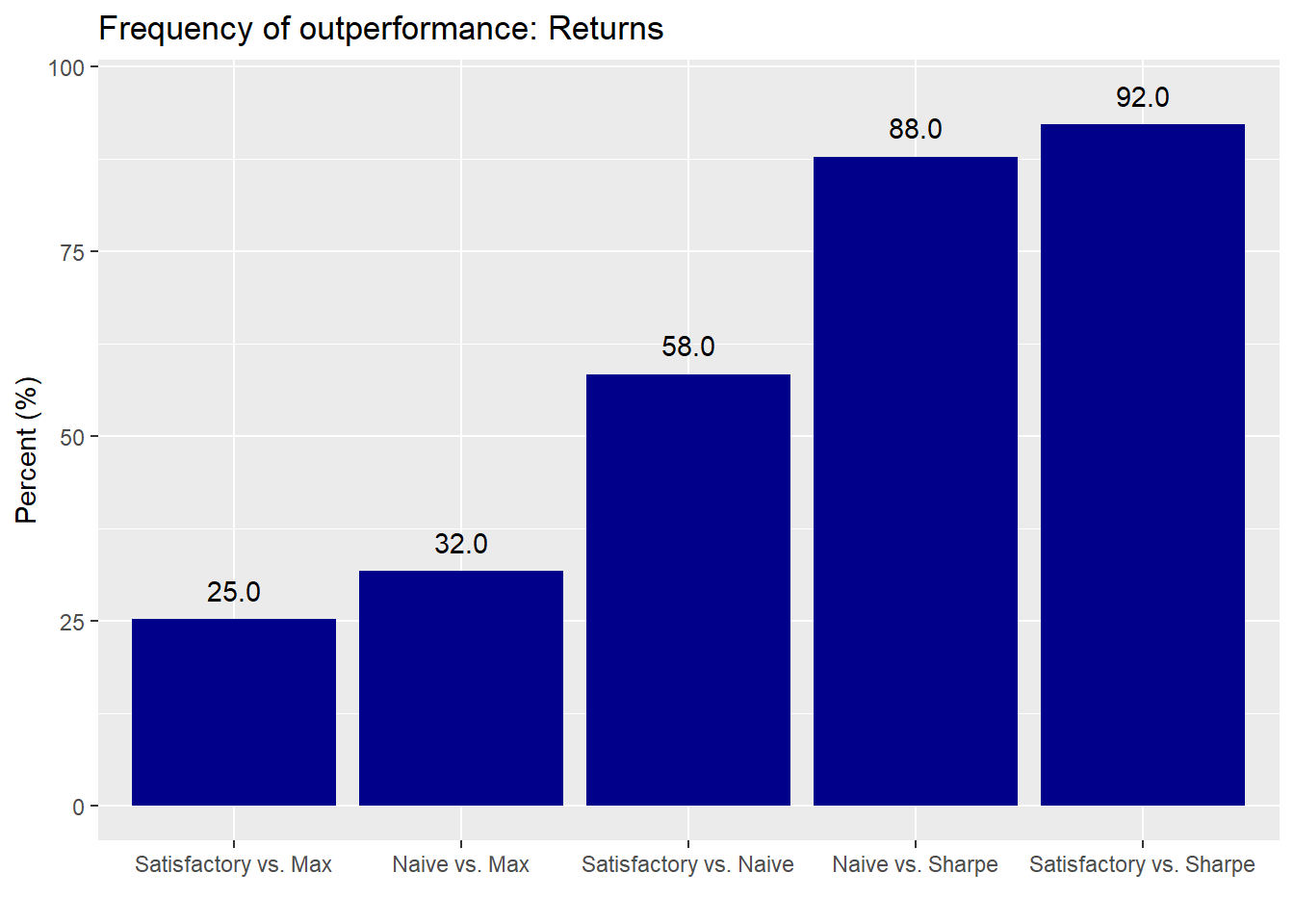Despite the 2% point outperformance of the maximum return portfolio relative to the satisfactory and naive portfolios, the frequency of outperformance suggests there could be a fair amount of randomness in that result. For example, the satisfactory and naive portfolios outperform the maximum return portfolio 25% and 32% of the time. There appears to be no significant difference between the satisfactory and naive portfolios since the frequency of outperformance is little better than a coin flip. And even though frequency of outperformance vs the highest Sharpe ratio portfolio is much higher for the naive and satisfactory portfolios relative to the others, it’s still below the 95% level most folks use to claim significance. That said, the satisfactory portfolio’s performance relative to the Sharpe portfolio is close to significant.

How do these portfolios perform in terms of risk-adjusted returns? Want to bet the Sharpe portfolio does well?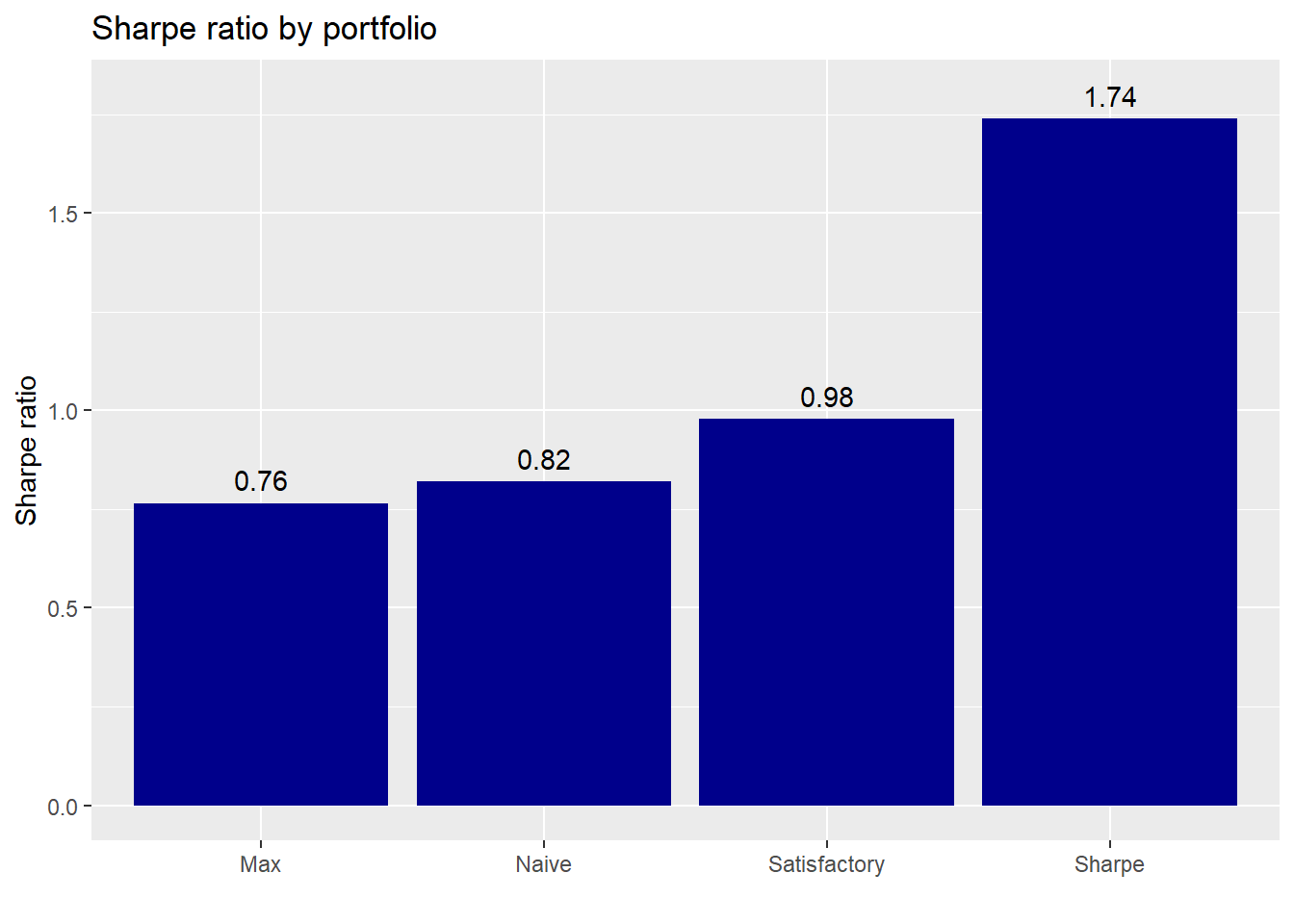Indeed, the Sharpe portfolio enjoys the highest risk-adjusted return on average for all the simulations, while the maximum return portfolio exhibits the lowest, a reversal of the average return performance. Let’s see how significant these results are.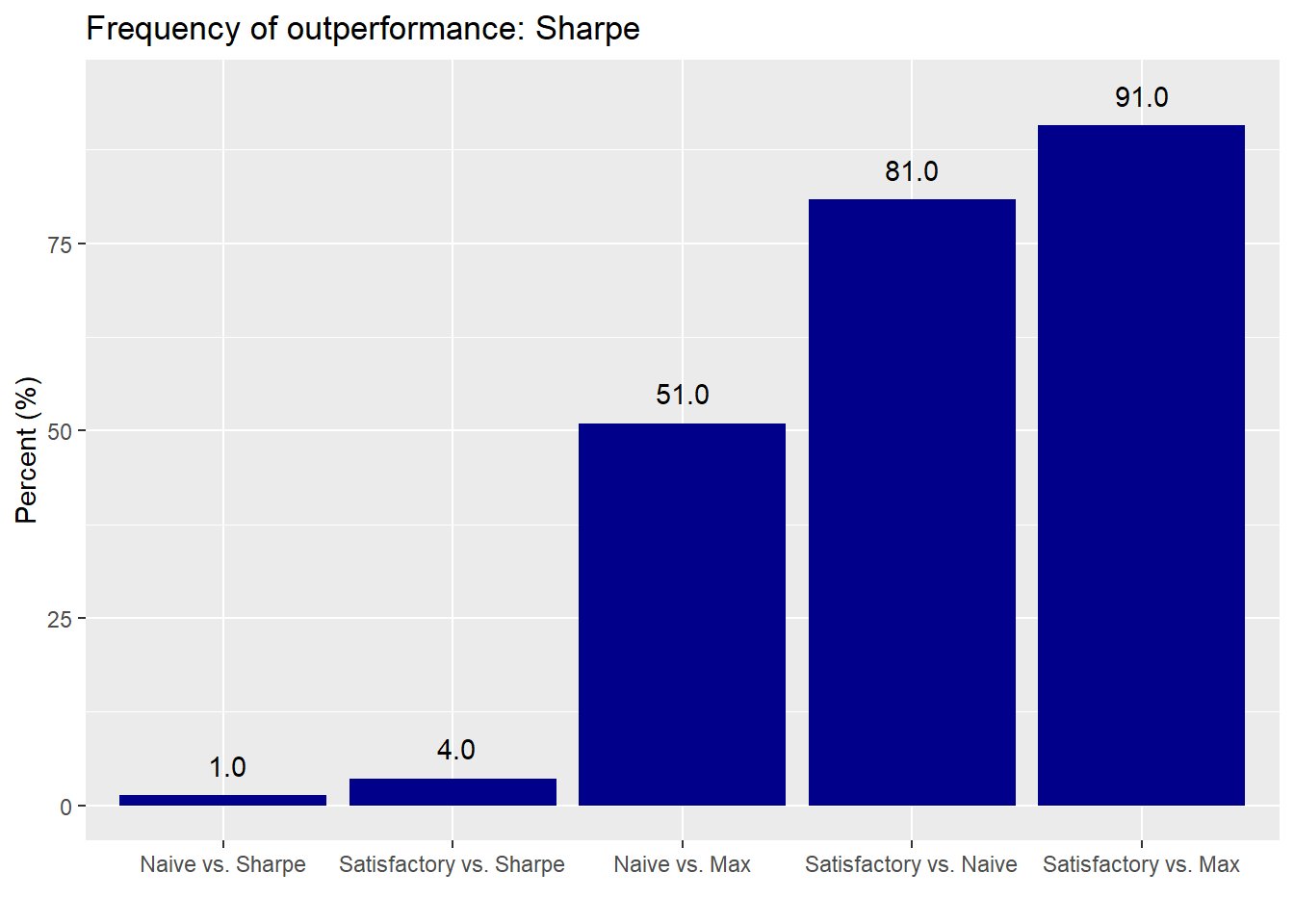The Sharpe portfolio’s performance definitely looks significant given that the satisfactory and naive portfolios only post better risk-adjusted returns 4% and 1% of the time. The naive portfolio’s Sharpe ratio is not meaningfully different than the maximum return portfolio, which is a bit surprising. While the satisfactory portfolio’s performance relative to the maximum portfolio might not meet the conventional 95% confidence hurdle to be significant, we would argue it’s close enough.

It’s good to see how each of the portfolio performs relative to the others, but what about relative to our simulation of three million portfolios from Weighting on a friend?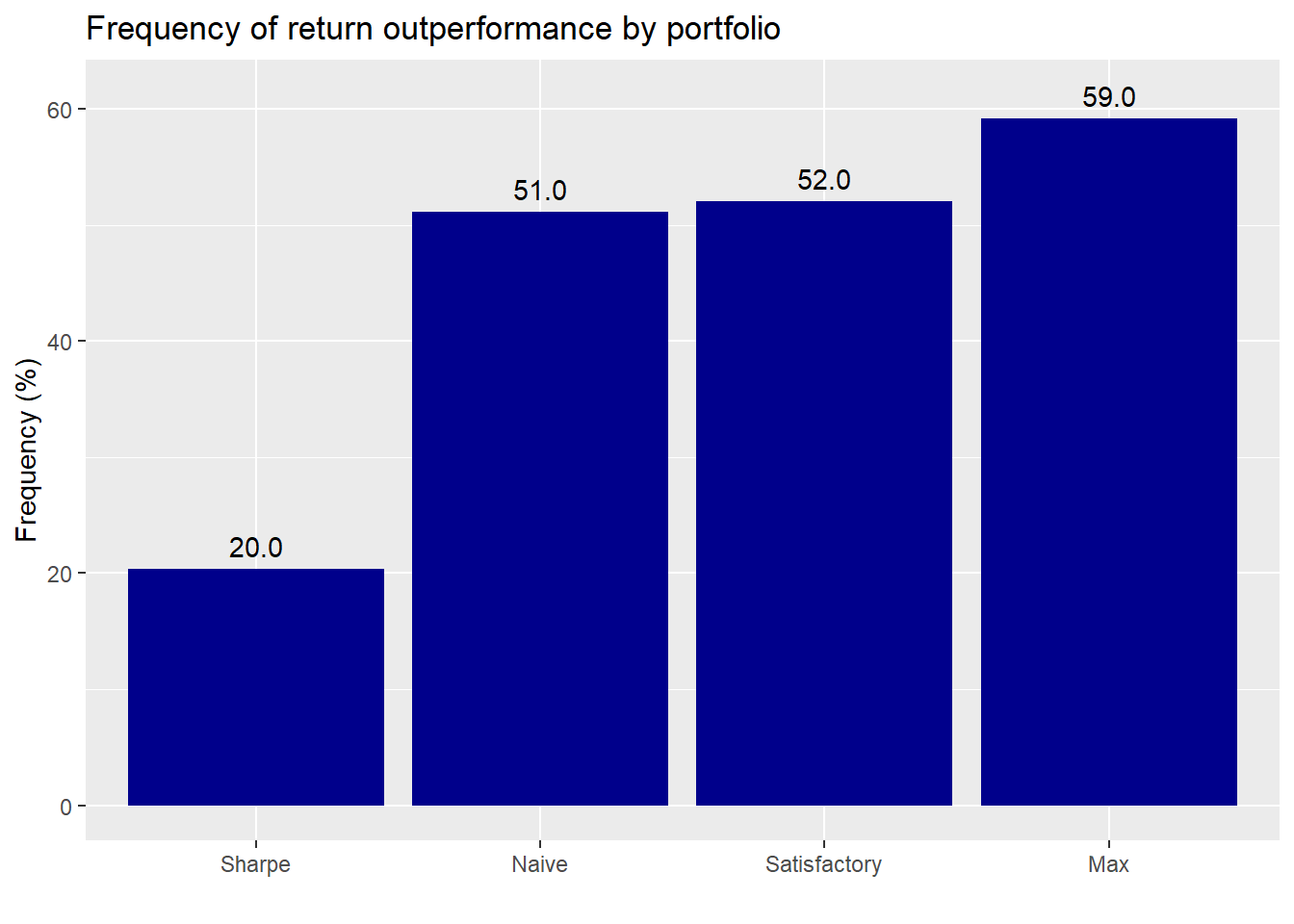Except for the Sharpe, most of the portfolios perform modestly better than the simulated portfolios. But the amount suggests mostly randomness. Surprisingly, the Sharpe portfolio performs quite poorly; we would not have expected 80% of the simulated portfolios to perform better. Let’s see how the portfolios compare on risk-adjusted returns.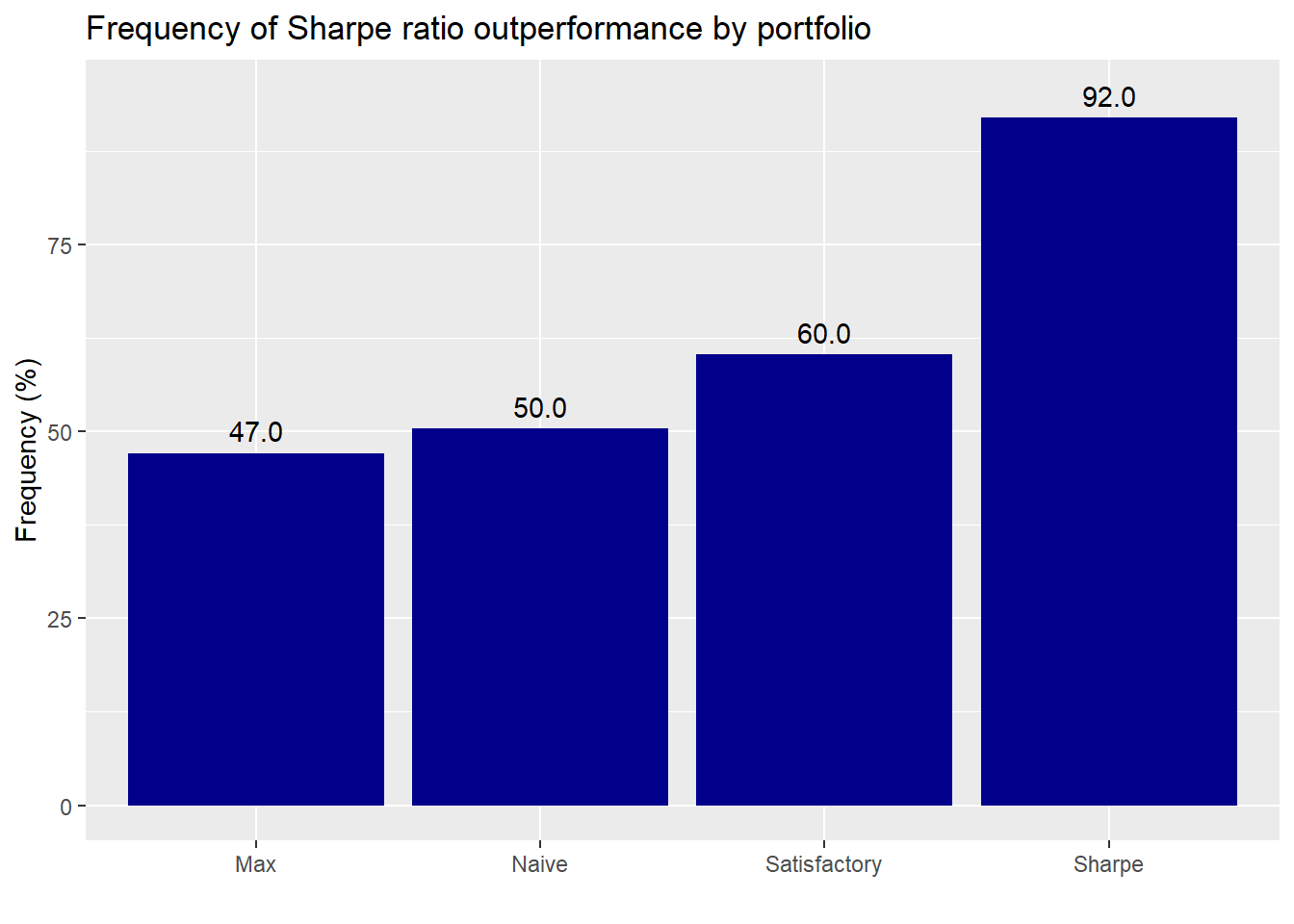The Sharpe portfolio outperforms close to a statistically significant level. The satisfactory portfolio’s performance isn’t bad. But the max and naive portfolios are coin tosses.

What are the key takeaways based on these results? You can shoot for high returns or high risk-adjusted returns, but rarely both. Assuming no major change in the underlying average returns and risk, choosing the efficient high return or high risk-adjusted return portfolio generally leads to similar performance a majority of the time in out-of-sample simulations. But such results are not necessarily statistically significant using conventional thresholds nor are they “efficient”. How the efficient portfolios would fare under different conditions remains an open question. But we suspect allowing for regime changes in average returns and risk would alter the performance significantly. While the naive portfolio wasn’t much of a star, it wasn’t that bad either. We suspect that if we had removed gold, the naive portfolio might have performed in line with the satisfactory.

While we weren’t arguing in favor of satisficing over optimizing, we see that a little bit of logic and some data science produced a solid relative performance. The satisfactory portfolio generally performed well on all metrics. Indeed, it rarely underperformed, and frequently outperformed the naive portfolio. Maybe the “good enough” naive portfolio could be a little better. Still, this doesn’t mean that one should give up on optimization, but it does suggest that you don’t need it to construct an acceptable portfolio. Of course, for the non-professional the amount of time required to achieve the data science skills and intuition to build the satisficing portfolio might be no different than the time required to understand and implement optimization. However, we beileve most folks can wrap their heads around the results produced by data science methods rather than optimization.

What did we miss in these analyses? We didn’t allow our algorithms to “learn”. That is, we didn’t update our allocations based on the simulations. For example, as we ran through each simulation we could have incorporated the returns from prior simulations to change the allocations. Or we could have randomized the sequence of simulations. True, there was no specific order to the simulations, but randomizing the sequence could introduce some path dependence that might produce interesting results. Needless to say, the naive results would still be the same since they are, after all, naive.

We also didn’t test cumulative returns over a sequence of a few simulations. This would be closer to the time horizon for most portfolios. And we didn’t allow for a change in the two “moments” that describe the distributions used in the simulations: namely the mean and standard deviation return. Lots more to investigate!

In the meantime, let us know what you think about the satisficing portfolio vis a vis the optimal one at our email address at the end of the code. Speaking of code, the Python and R versions are below.

#### Python code

```# Built using Python 3.7.4

import pandas as pd
import pandas_datareader.data as web
import numpy as np
import matplotlib.pyplot as plt
%matplotlib inline

import Port_sim # for class and methods see prior po  sts

plt.style.use('ggplot')

# Calculate returns and risk for longer period
hist_mu = dat['1971':'1991'].mean(axis=0)
hist_sigma = dat['1971':'1991'].std(axis=0)

# Run simulation based on historical figures
np.random.seed(123)
sim1 = []

for i in range(1000):
#np.random.normal(mu, sigma, obs)
a = np.random.normal(hist_mu, hist_sigma, 60) + np.random.normal(0, hist_sigma, 60)
b = np.random.normal(hist_mu, hist_sigma, 60) + np.random.normal(0, hist_sigma, 60)
c = np.random.normal(hist_mu, hist_sigma, 60) + np.random.normal(0, hist_sigma, 60)
d = np.random.normal(hist_mu, hist_sigma, 60) + np.random.normal(0, hist_sigma, 60)

df1 = pd.DataFrame(np.array([a, b, c, d]).T)

cov_df1 = df1.cov()

sim1.append([df1, cov_df1])

# Create portfolio simulation
np.random.seed(123)
port_sim_1, wts_1, _, sharpe_1, _ = Port_sim.calc_sim(df.iloc[1:60,0:4],1000,4)

# Create efficient frontier function
from scipy.optimize import minimize

def eff_frontier(df_returns, min_ret, max_ret):

n = len(df_returns.columns)

def get_data(weights):
weights = np.array(weights)
returns = np.sum(df_returns.mean() * weights)
risk = np.sqrt(np.dot(weights.T, np.dot(df_returns.cov(), weights)))
sharpe = returns/risk
return np.array([returns,risk,sharpe])

# Contraints
def check_sum(weights):
return np.sum(weights) - 1

# Rante of returns
mus = np.linspace(min_ret,max_ret,21)

# Function to minimize
def minimize_volatility(weights):
return  get_data(weights)

# Inputs
init_guess = np.repeat(1/n,n)
bounds = ((0.0,1.0),) * n

eff_risk = []
port_weights = []

for mu in mus:
# function for return
cons = ({'type':'eq','fun': check_sum},
{'type':'eq','fun': lambda w: get_data(w) - mu})

result = minimize(minimize_volatility,init_guess,method='SLSQP',bounds=bounds,constraints=cons)

eff_risk.append(result['fun'])
port_weights.append(result.x)

eff_risk = np.array(eff_risk)

return mus, eff_risk, port_weights

# Create returns and min/max ranges
df_returns = df.iloc[1:60, 0:4]
min_ret = min(port_sim_1[:,0])
max_ret = max(port_sim_1[:,0])

# Find efficient portfolio
eff_ret, eff_risk, eff_weights = eff_frontier(df_returns, min_ret, max_ret)
eff_sharpe = eff_ret/eff_risk

### Test results of different weighting schemes on simulated returns
## Create weight schemes
satisfice_wts = np.array([0.32, 0.4, 0.08, 0.2]) # Calculated in previous post using port_select_func
simple_wts = np.repeat(0.25, 4)
eff_sharp_wts = eff_weights[np.argmax(eff_sharpe)]
eff_max_wts = eff_weights[np.argmax(eff_ret)]

## Create portfolio metric function to iterate
def port_func(df, wts):
mean_ret = df.mean()
returns = np.sum(mean_ret * wts)
risk = np.sqrt(np.dot(wts, np.dot(df.cov(), wts)))
return returns, risk

# Run portfolio returns for return simulations
from datetime import datetime
start_time = datetime.now()

list_df = [np.zeros((1000,2)) for _ in range(4)]
wt_list = [satisfice_wts, simple_wts, eff_sharp_wts, eff_max_wts]

for i in range(4):
arr = list_df[i]
for j in range(1000):
arr[j] = port_func(sim1[j], wt_list[i])

sharpe_calc = arr[:,0]/arr[:,1]
list_df[i] = np.c_[arr, sharpe_calc]

satis_df = list_df
simple_df = list_df
eff_sharp_df = list_df
eff_max_df = list_df

end_time = datetime.now()
print('Duration: {}'.format(end_time - start_time))

# Note python produces this much faster than R. Duration: 0:00:03.226398. Our R code must not be optimized.

# Create portfolio means and names for graphing

port_means = []

for df in list_df:
port_means.append(df[:][:,0].mean()*1200)

port_names = ['Satisfactory','Naive', 'Sharpe', 'Max']

# Create graphing function

def pf_graf(names, values, rnd, nudge, ylabs, graf_title):
df = pd.DataFrame(zip(names, values), columns = ['key', 'value'])
sorted = df.sort_values(by = 'value')
plt.figure(figsize = (12,6))
plt.bar('key', 'value', data = sorted, color='darkblue')

for i in range(len(names)):
plt.annotate(str(round(sorted['value'][i], rnd)), xy = (sorted['key'][i], sorted['value'][i]+nudge))

plt.ylabel(ylabs)
plt.title('{} performance by portfolio'.format(graf_title))
plt.show()

# Graph return performance by portfolio
pf_graf(port_names, port_means, 2, 0.5, 'Returns (%)', 'Return')

# Build names for comparison chart
comp_names= []
for i in range(4):
for j in range(i+1,4):
comp_names.append('{} vs. {}'.format(port_names[i], port_names[j]))

# Calculate comparison values
comp_values = []

for i in range(4):
for j in range(i+1, 4):
comps =np.mean(list_df[i][:][:,0] > list_df[j][:][:,0])
comp_values.append(comps)

# Graph comparisons
pf_graf(comp_names[:-1], comp_values[:-1], 2, 0.025, 'Frequency (%)', 'Frequency of')

# Build Sharpe portfolio comparisons

sharp_means = []
for df in list_df:
sharp_means.append(df[:][:,2].mean()*np.sqrt(12))

sharp_comp = []
for i in range(4):
for j in range(i+1, 4):
comp = np.mean(list_df[i][:][:,2] > list_df[j][:][:,2])
sharp_comp.append(comp)

# Graph mean return comparsions for sharpe porfolio
pf_graf(port_names, sharp_means, 2, 0.005, "Sharpe ratio", "Sharpe ratio")

# Graph sharpe results for sharpe portoflio
pf_graf(comp_names[:-1], sharp_comp[:-1], 2, 0.005, "Frequency(%)", "Frequency")

# Bring in port simulation to compare results across million portfolios
sharpe_1m = port_1m[:,0]/port_1m[:,1]

# Create mean and sharpe outperformance results lists
sim_mean = []
sim_sharp = []

for i in range(4):
mean = np.mean(np.mean(list_df[i][:,0]) > port_1m[:,0])
sim_mean.append(mean)
sharp = np.mean(np.mean(list_df[i][:,2]) > sharpe_1m[:])
sim_sharp.append(sharp)

# Graph return outperformance
pf_graf(port_names, sim_mean, 2, 0.005, "Frequency(%)", "Frequency")

# Graph sharpe outperformance
pf_graf(port_names, sim_sharp, 2, 0.005, 'Frequency (%)', 'Frequency')```

#### R code

```# Built using R 3.6.2

suppressPackageStartupMessages({
library(tidyquant)
library(tidyverse)
})

## Load data. see prior posts for code that produced data
sym_names <- c("stock", "bond", "gold", "realt", "rfr")

## Call functions. See prior posts for functions
source("Portfolio_simulation_functions.R")
source("Efficient_frontier.R")

## Prepare sample
hist_avg <- dat %>%
filter(date <= "1991-12-31") %>%
summarise_at(vars(-date), list(mean = function(x) mean(x, na.rm=TRUE),
sd = function(x) sd(x, na.rm = TRUE))) %>%
gather(key, value) %>%
mutate(key = str_remove(key, "_.*"),
key = factor(key, levels =sym_names)) %>%
mutate(calc = c(rep("mean",5), rep("sd",5))) %>%

# Run simulation
set.seed(123)
sim1 <- list()
for(i in 1:1000){
a <- rnorm(60, hist_avg[1,2], hist_avg[1,3]) + rnorm(60, 0, hist_avg[1,3])
b <- rnorm(60, hist_avg[2,2], hist_avg[2,3]) + rnorm(60, 0, hist_avg[2,3])
c <- rnorm(60, hist_avg[3,2], hist_avg[3,3]) + rnorm(60, 0, hist_avg[3,3])
d <- rnorm(60, hist_avg[4,2], hist_avg[4,3]) + rnorm(60, 0, hist_avg[4,3])

df1 <- data.frame(a, b, c, d)

cov_df1 <- cov(df1)

sim1[[i]] <- list(df1, cov_df1)

names(sim1[[i]]) <- c("df", "cov_df")

}

### Test results of different weighting schemes on simulated returns
## Create weight schemes
satis_wts <- c(0.32, 0.4, 0.08, 0.2) # Calculated in previous post using port_select_func
simple_wts <- rep(0.25, 4)
eff_port <- eff_frontier_long(df[2:61,2:5], risk_increment = 0.01)
eff_sharp_wts <- eff_port[which.max(eff_port\$sharpe),1:4] %>% as.numeric()
eff_max_wts <- eff_port[which.max(eff_port\$exp_ret), 1:4] %>% as.numeric()

## Test run with port sim on sample 12
port_sim_1 <- port_sim_lv(sim1[]\$df,1000,4)
eff_front_sim_1 <- eff_frontier_long(sim1[]\$df)

## Creater function to iterate
port_func <- function(df,wts){
mean_ret = colMeans(df)
returns = sum(mean_ret*wts)
risk = sqrt(t(wts) %*% cov(df) %*% wts)
c(returns, risk)
}

# Run function on three weighting schemes and one simulation
weight_list <- list(satis = satis_wts,
naive = simple_wts,
sharp = eff_sharp_wts,
max = eff_max_wts)

wts_df <- data.frame(wts = c("satis", "naive", "sharp", "max"), returns = 1:4, risk = 5:8,
stringsAsFactors = FALSE)
for(i in 1:4){
wts_df[i, 2:3] <- port_func(sim1[]\$df, weight_list[[i]])

}

wts_df\$sharpe = wts_df\$returns/wts_df\$risk

# Graph portfolio simulation with three portfolios
port_sim_1\$graph +
geom_point(data = wts_df,
aes(x = risk*sqrt(12)*100, y = returns*1200),
color = c("darkblue", "black", "red", "red"),
size = 4) +
geom_line(data = eff_port,
aes(stdev*sqrt(12)*100, exp_ret*1200),
color = 'blue',
size = 1.5) +
theme(legend.position = c(0.05,0.8), legend.key.size = unit(.5, "cm"),
legend.background = element_rect(fill = NA))

# Calculate metrics based on weighting scenario
dfs = c("satis_df", "simple_df", "eff_df", "eff_ret_df")

for(i in 1:4){
assign(dfs[i], port_18_pfs[[i]])
}

Create portfolio data frames
dfs = c("satis_df", "simple_df", "eff_df", "eff_ret_df")
wts_list = list(satisfice_wts, simple_wts, eff_wts, eff_ret_wts)

for(i in 1:4){
x <- lapply(seq_along(sim1), function(y) rebal_ret(sim1[[y]]\$df, wts_list[[i]]))
x <- do.call("rbind", x)
x <- x %>%
as.data.frame() %>%
`colnames<-`(c("returns", "risk")) %>%
mutate(sharpe = returns/risk)
assign(dfs[i], x)
}

# create mean portfolio return graph
pf_graf <- function(df, nudge, multiplier, rnd, y_lab, text){
df %>%
gather(key, value)  %>%
ggplot(aes(reorder(key, value), value*multiplier*100)) +
geom_bar(stat='identity',
fill = 'darkblue') +
geom_text(aes(label = format(round(value*multiplier,rnd)*100,nsmall = 1)), nudge_y = nudge)+
labs(x = "",
y = paste(y_lab, "(%)", sep = " "),
title = paste(text, "by portfolio", sep = " "))
}

# Create mean return data frame
mean_pf <- data.frame(Satisfactory = mean(satis_df[,1]),
Naive = mean(simple_df[,1]),
Sharpe = mean(eff_df[,1]),
Max = mean(eff_ret_df[,1]))

# Graph mean returns
pf_graf(mean_pf, 1, 12, 2,"Return")

## Calculate outperformance
# Create outperformance graph
perf_graf <- function(df, nudge, text){
df %>%
rename("Satisfactory vs. Naive" = ovs,
"Satisfactory vs. Max" = ovr,
"Naive vs. Max" = rve,
"Satisfactory vs. Sharpe" = ove,
"Naive vs. Sharpe" = sve) %>%
gather(key, value)  %>%
ggplot(aes(reorder(key, value), value*100)) +
geom_bar(stat='identity',
fill = 'darkblue') +
geom_text(aes(label = round(value,2)*100), nudge_y = nudge)+
labs(x = "",
y = "Percent (%)",
title = paste("Frequency of outperformance:", text, sep = " "))

}

# Create performance data frame
# Return
ret_pf <- data.frame(ovs = mean(satis_df[,1] > simple_df[,1]),
ovr = mean(satis_df[,1] > eff_ret_df[,1]),
rve = mean(simple_df[,1] > eff_ret_df[,1]),
ove = mean(satis_df[,1] > eff_df[,1]),
sve = mean(simple_df[,1] > eff_df[,1]))

# Graph outperformance
perf_graf(ret_pf, 4, "Returns")

# Sharpe portfolios
sharp_port <- data.frame(Satisfactory = mean(satis_df[,3]),
Naive = mean(simple_df[,3]),
Sharpe = mean(eff_df[,3]),
Max = mean(eff_ret_df[,3]))

pf_graf(sharp_port, 6, sqrt(12), 2,"Sharpe ratio")

sharp_pf <- data.frame(ovs = mean(satis_df[,3] > simple_df[,3]),
ovr = mean(satis_df[,3] > eff_ret_df[,3]),
rve = mean(simple_df[,3] > eff_ret_df[,3]),
ove = mean(satis_df[,3] > eff_df[,3]),
sve = mean(simple_df[,3] > eff_df[,3]))

perf_graf(sharp_pf, 4, "Sharpe")

## Simulated portfolios

# Call port_1m

port_1m\$sharpe <- port_1m\$returns/port_1m\$risk
sim_mean <- data.frame(Satisfactory = mean(satis_df[,1] > port_1m[,1]),
Naive = mean(simple_df[,1] > port_1m[,1]),
Sharpe = mean(eff_df[,1] > port_1m[,1]),
Max = mean(eff_ret_df[,1] > port_1m[,1]))

sim_sharp <- data.frame(Satisfactory = mean(satis_df[,3] > port_1m[,3]),
Naive = mean(simple_df[,3] > port_1m[,3]),
Sharpe = mean(eff_df[,3] > port_1m[,3]),
Max = mean(eff_ret_df[,3] > port_1m[,3]))

# Graph return outperformance
pf_graf(sim_mean, 2, 1, 2, "Frequency", "Frequency of return outperformance")

# Graph Sharpe outperformance
pf_graf(sim_sharp, 3, 1, 2, "Frequency", "Frequency of Sharpe ratio outperformance")```

1. It’s the tangency portfolio because if one were to draw a line from the risk-free rate on the return axis such that it’s tangent to the efficient frontier, that point would happen to be the portfolio with the highest Sharpe ratio. Note that the tangency portfolio does not necessarily offer the highest return since the efficient frontier is a sideways parabola and the risk-free rate “shouldn’t” offer a higher expected return than riskier assets.